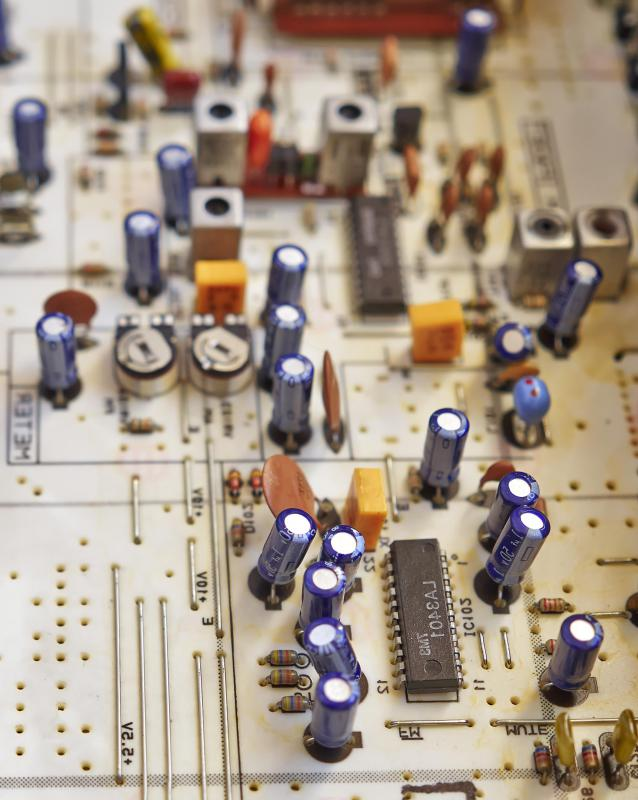# What is a Shunt Resistor?

Kevin Mathews

A shunt resistor is a precision device used to measure current in an electrical circuit. Also known as a current shunt or an ammeter shunt, it works by measuring the voltage drop across a known resistance. Ohm’s law states that V = I x R, or solving for I, I = V / R, where I is current, V is voltage, and R is resistance. If the resistance is known and the voltage drop is measured, then the current can be determined.A resistor is an electronic component that can lower a circuit’s voltage and its flow of electrical current.

Shunt resistors are used to measure currents that would potentially damage an ammeter. This could be a result of the magnitude of the current passing through the circuit or the possibility of current spikes. They usually have a small, well-defined resistance so as not to affect the current they are measuring. A shunt resistor typically looks different from a normal resistor, having two large terminals with one or more strips of metal connecting them. The resistance of a metal is inversely proportional to its cross-sectional area, so the more strips a shunt resistor has, the lower its resistance.

For example, a shunt resistor with a resistance of 0.001 ohm that reads a voltage drop of 0.02 volt has a 20-amp current flowing through it (0.02 / 0.001 = 20). This measurement is not exact, because it relies on the resistance being a strict and constant 0.001 ohm. For a couple reasons, this is not the case.

First, the resistance of the device itself has a margin of error. While an ideal shunt resistor in the above example would have precisely 0.001 ohm, in reality there is a margin of error called the resistance accuracy. Assuming it is +/- 0.25 percent would mean the measured current is between 19.95 and 20.05 ohms (+/- 0.0025 * 20 = +/- 0.05).

Second, current passing through a resistor produces heat. Heat changes the actual resistance of a shunt resistor. How much is determined by the device’s resistance drift, usually measured in parts per million (ppm) per degree of temperature change. For the resistor in the above example, assuming a 30 ppm per degree of temperature change and a 20° change in temperature would mean the measured current is between 19.988 and 20.012 ohms (+/- 30 ppm * 20 = +/- 0.012).

In addition to resistance accuracy and resistance drift, shunt resistors are also characterized by current rating and power rating. The current rating is the maximum amount of current that can pass through the shunt without damaging it. This current generates heat, which in turn affects the resistance of the shunt. The power rating is the maximum amount of current that can continuously pass through the shunt without damaging it or adversely affecting its resistance. In general, this is 2/3 of its current rating.

## You might also LikeGrinderry

I don't think there would be a way to generate enough electrical power for something on a global scale. Continental definitely, as a lot of the continents generate enough electricity to power various segments of their land mass, but global power generation would still be limited by distance, terrain, weather and other factors that make it almost impossible.

aageon

Can this be used on a larger scale? Larger than the conventional methods and means that it is used for now? Like taking these principles and concepts I wonder if this would be something that can be achieved on a continental or even global scale.

CrazyGamer6

I am constantly amazed at the power of electricity and the way it can alter both metal and just about any other material it comes into contact with. I wonder if there's ever been a time when a shunt resistor couldn’t hold up to the current traveling through it